# Selina Solutions Concise Maths Class 10 Chapter 10 Arithmetic Progression

An Arithmetic Progression is a sequence of numbers in which each term can be obtained by adding a certain quantity to its preceding term. This is an interesting topic to learn and is also one of the important chapters of ICSE Class 10 Mathematics. Students who are finding it difficult to solve problems of Selina Solutions for Class 10 Mathematics can download the PDF of solutions prepared by subject experts at BYJU’S who have a vast academic experience. Here, the students can access the Selina Solutions for Class 10 Mathematics Chapter 10 Arithmetic Progression free PDF to improve their speed in solving problems as well as increase their confidence before taking the final board exam.

## Selina Solutions Concise Maths Class 10 Chapter 10 Arithmetic Progression Download PDF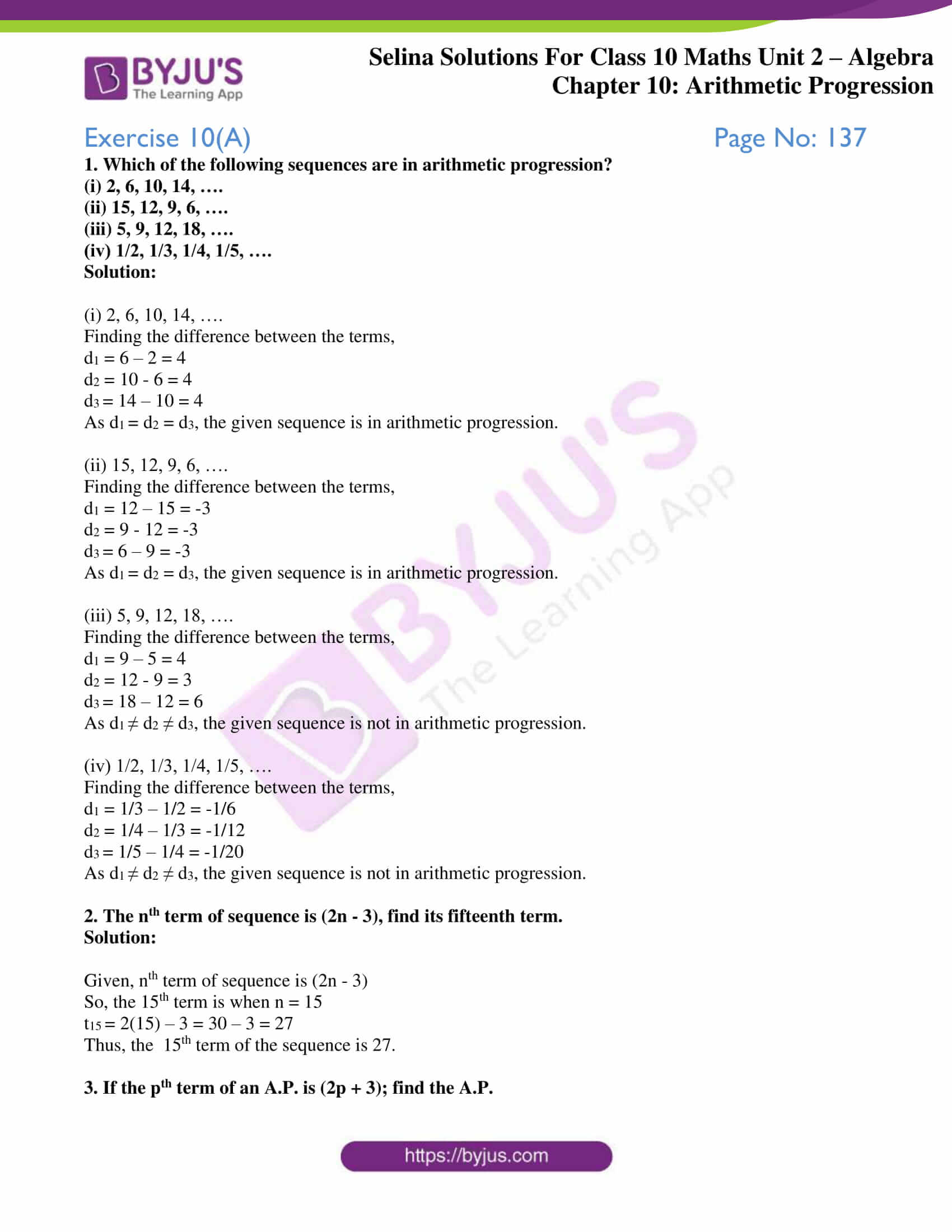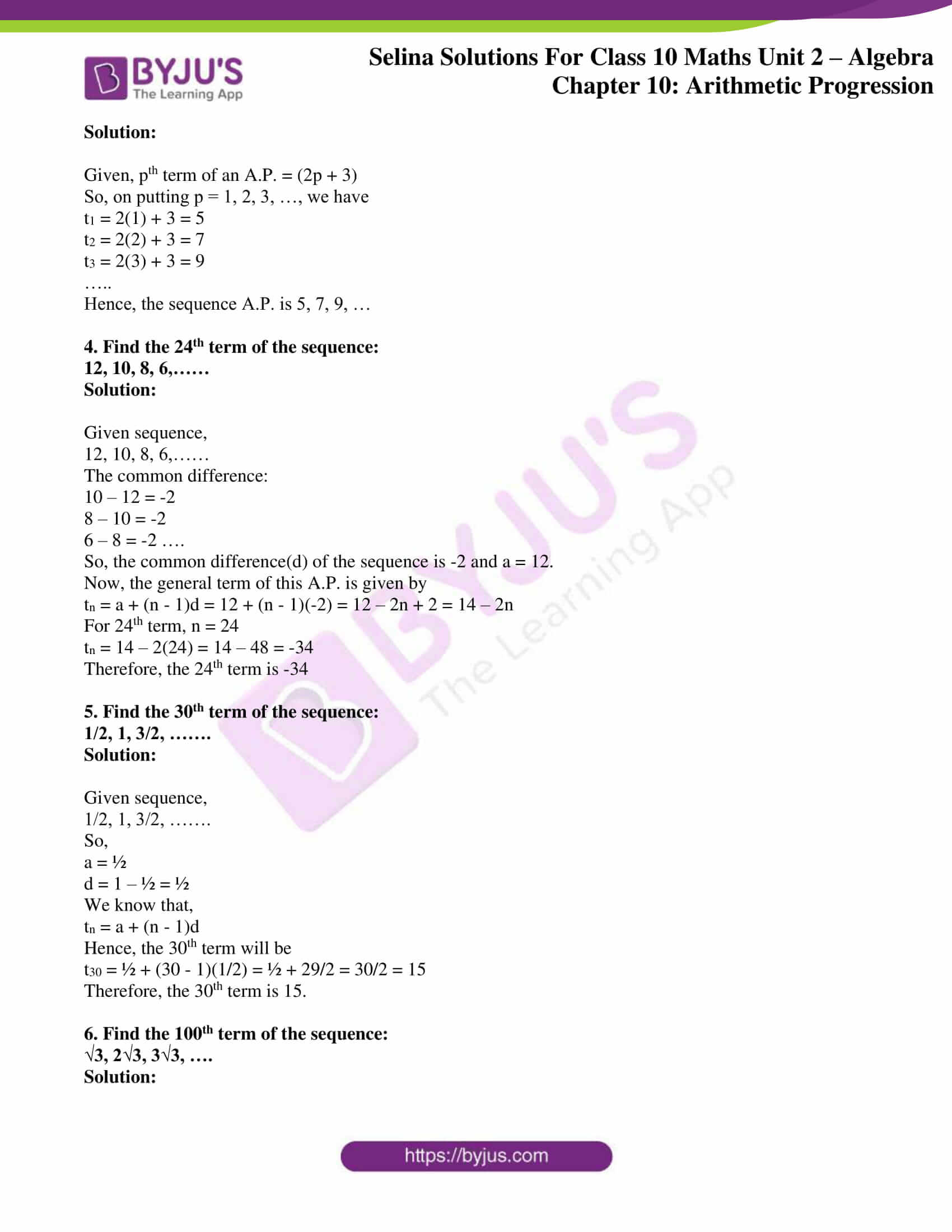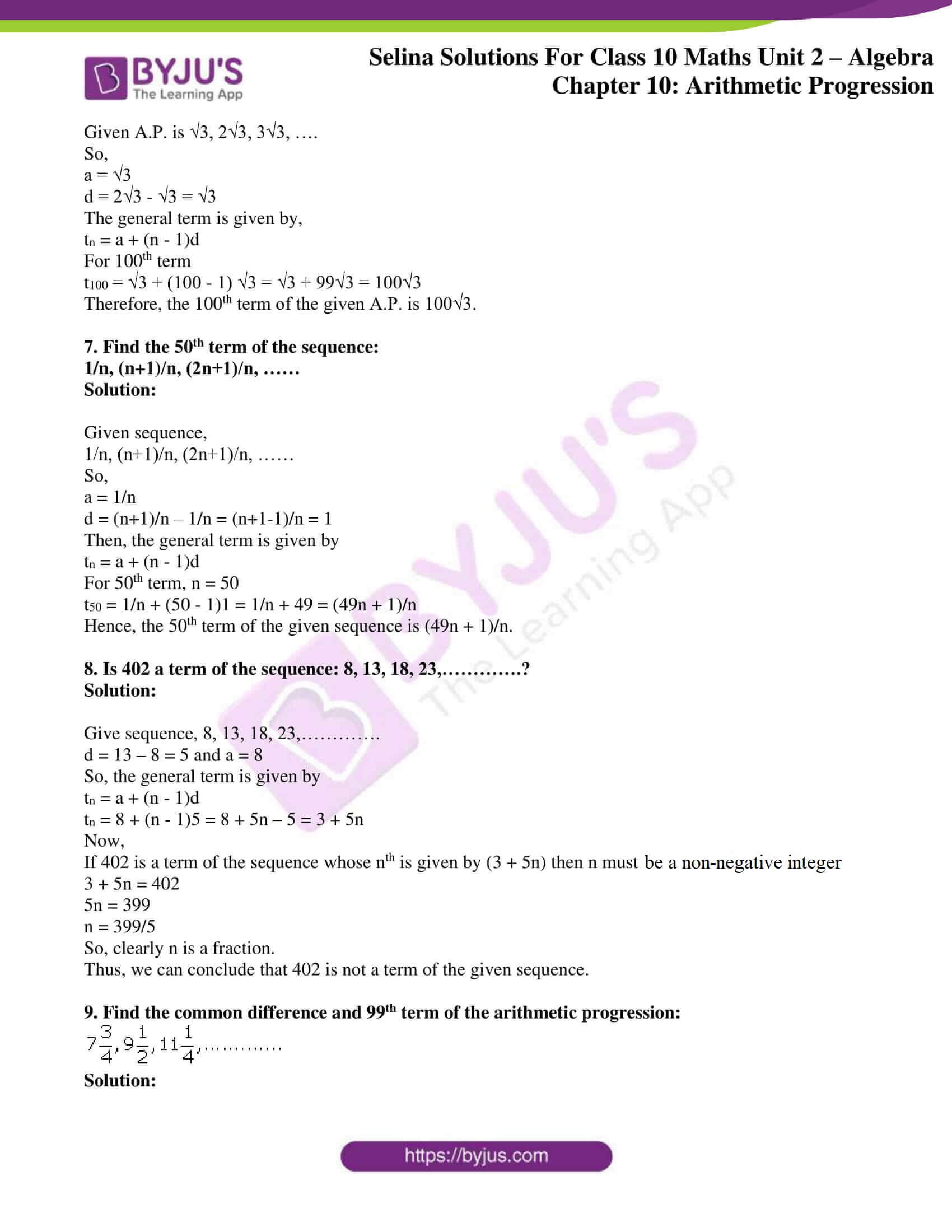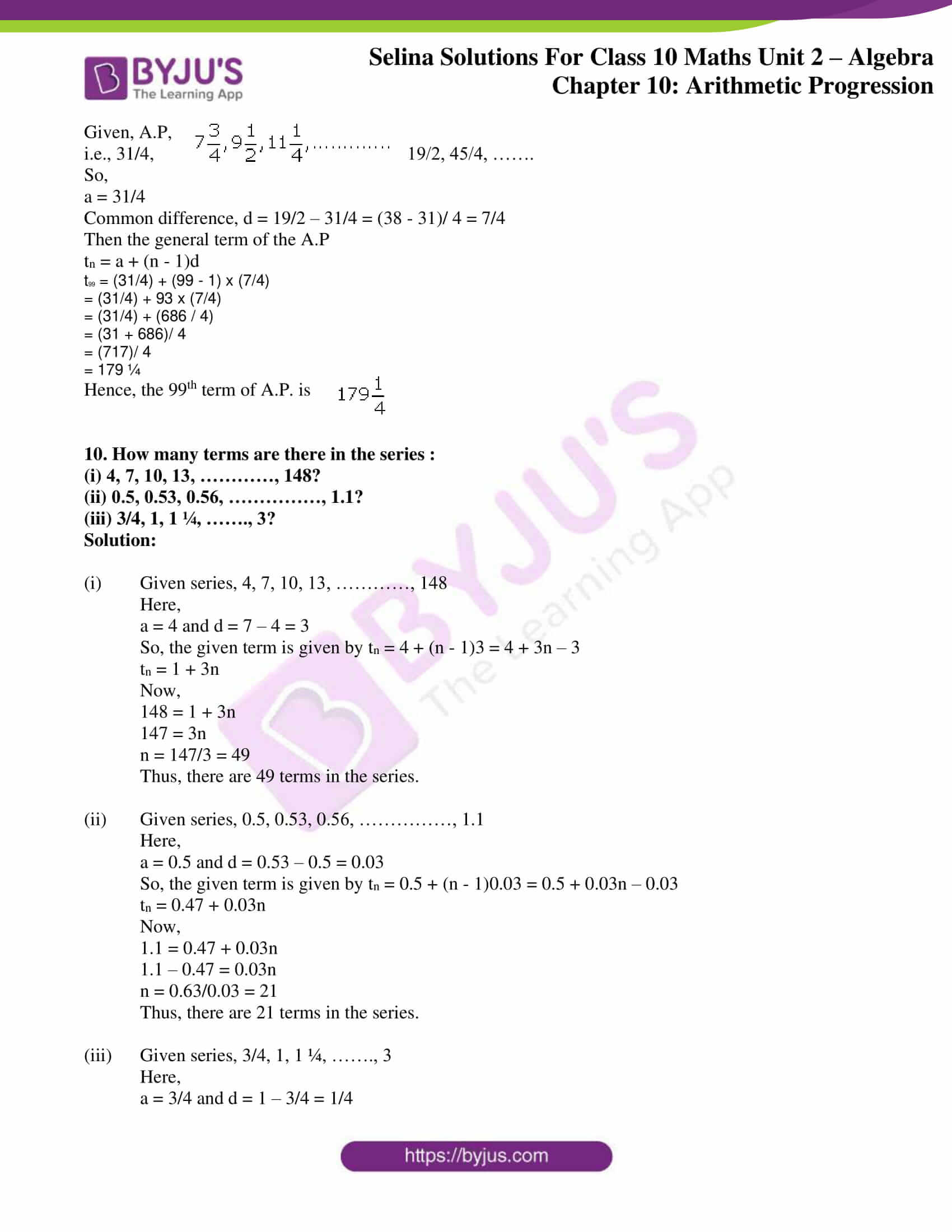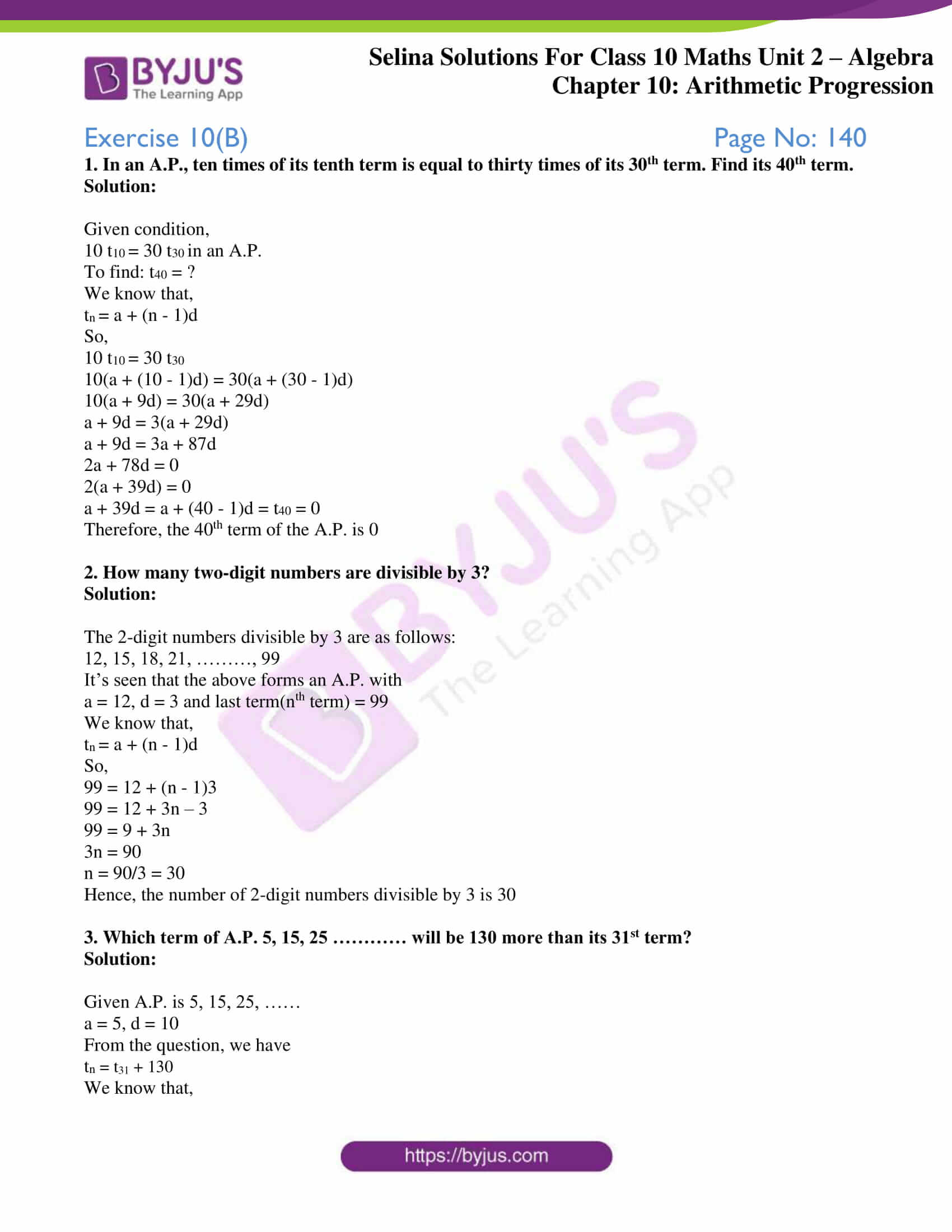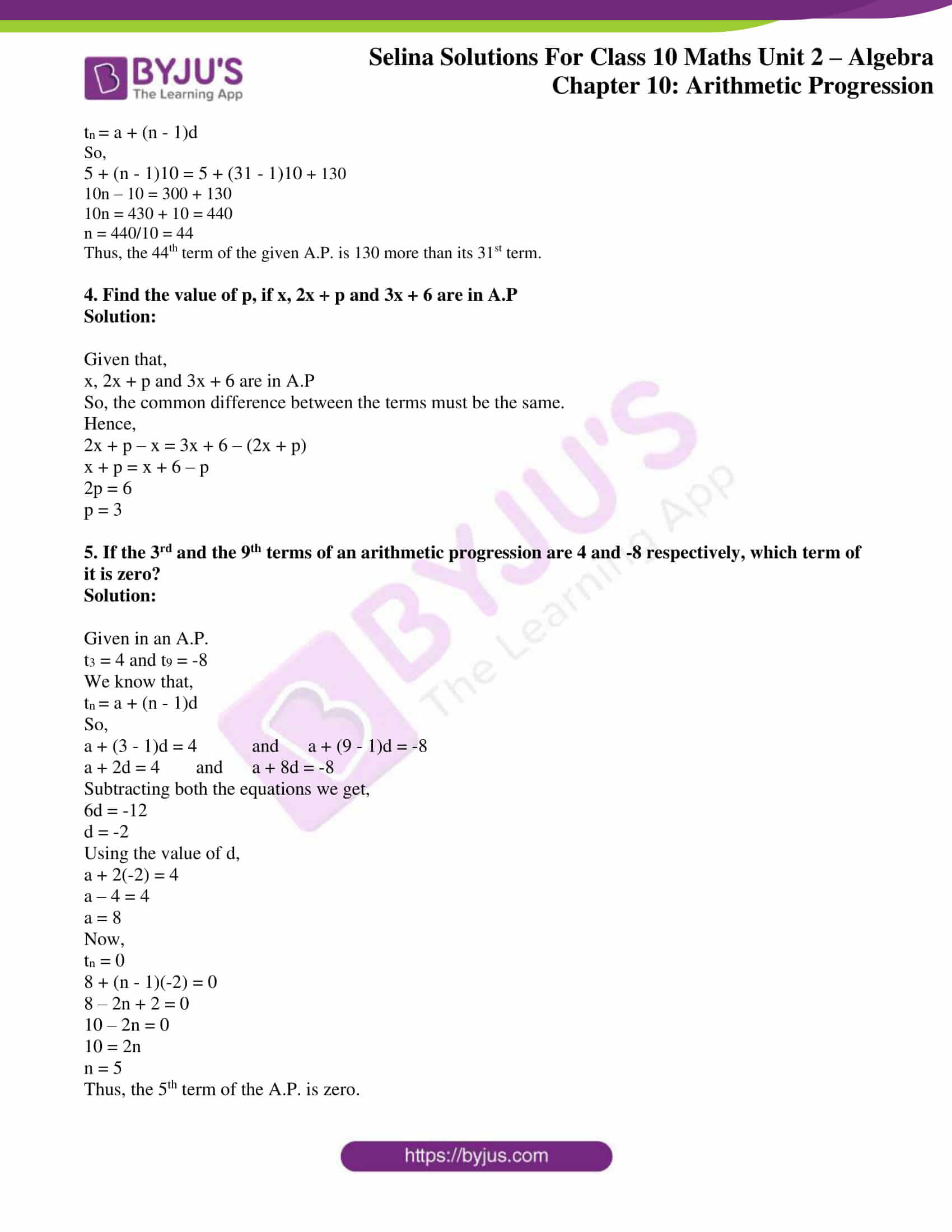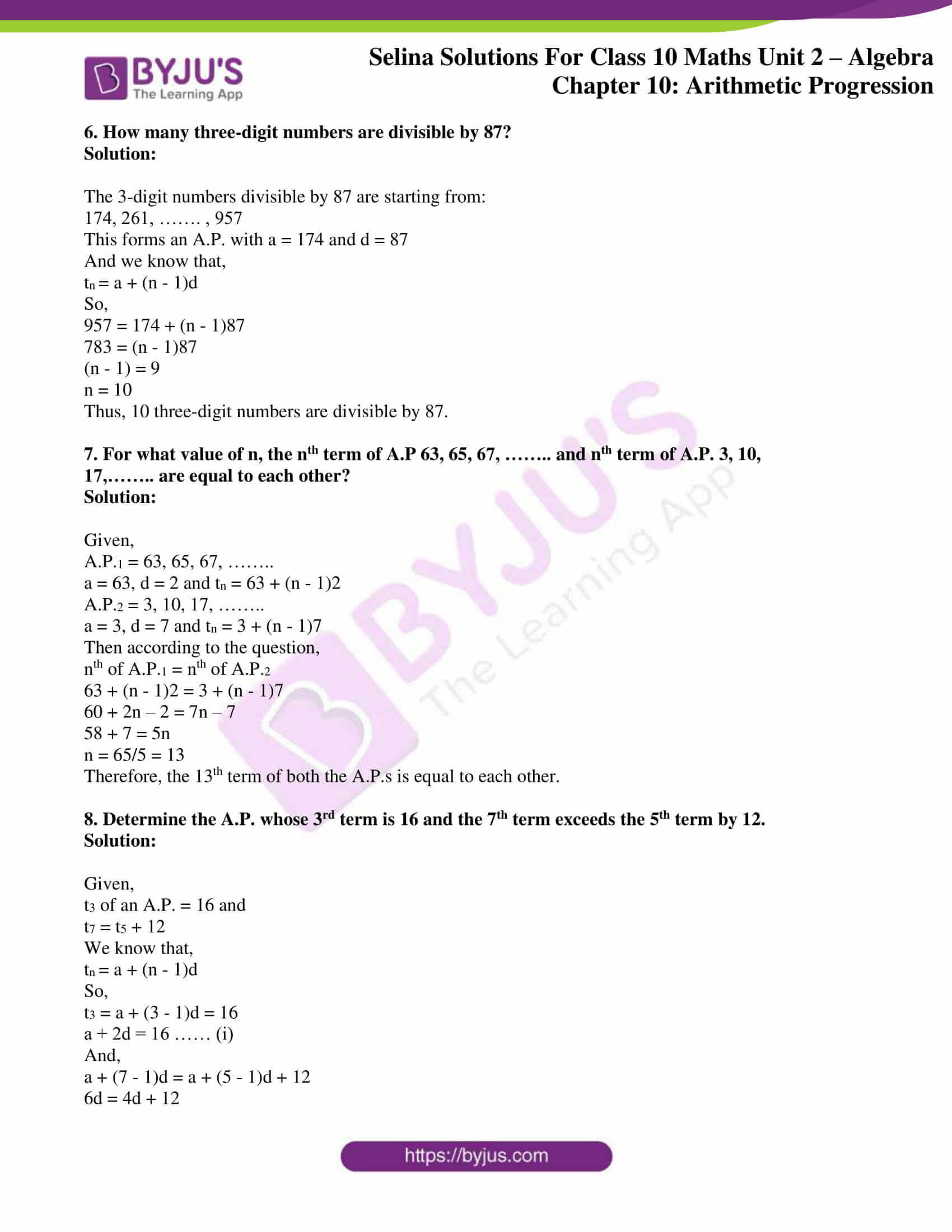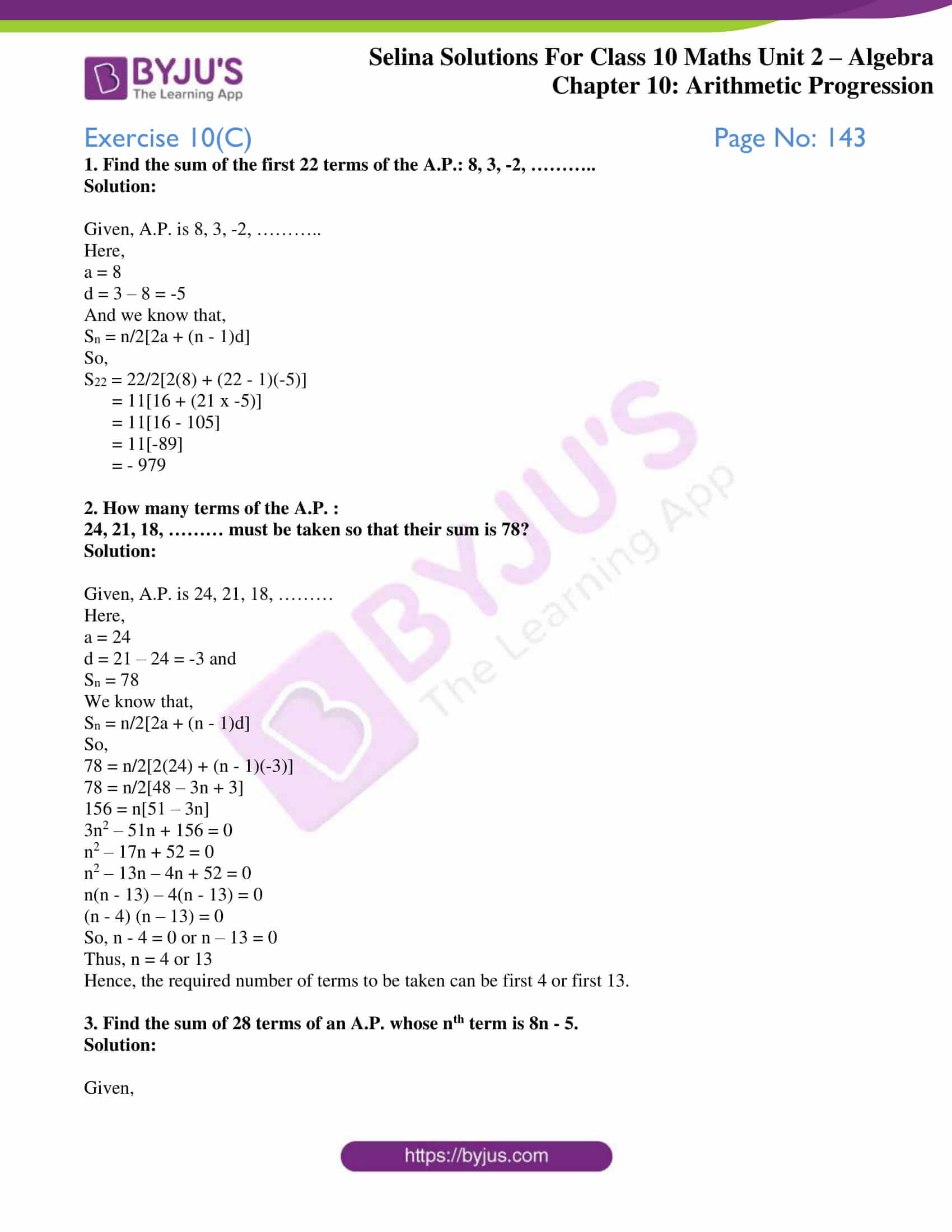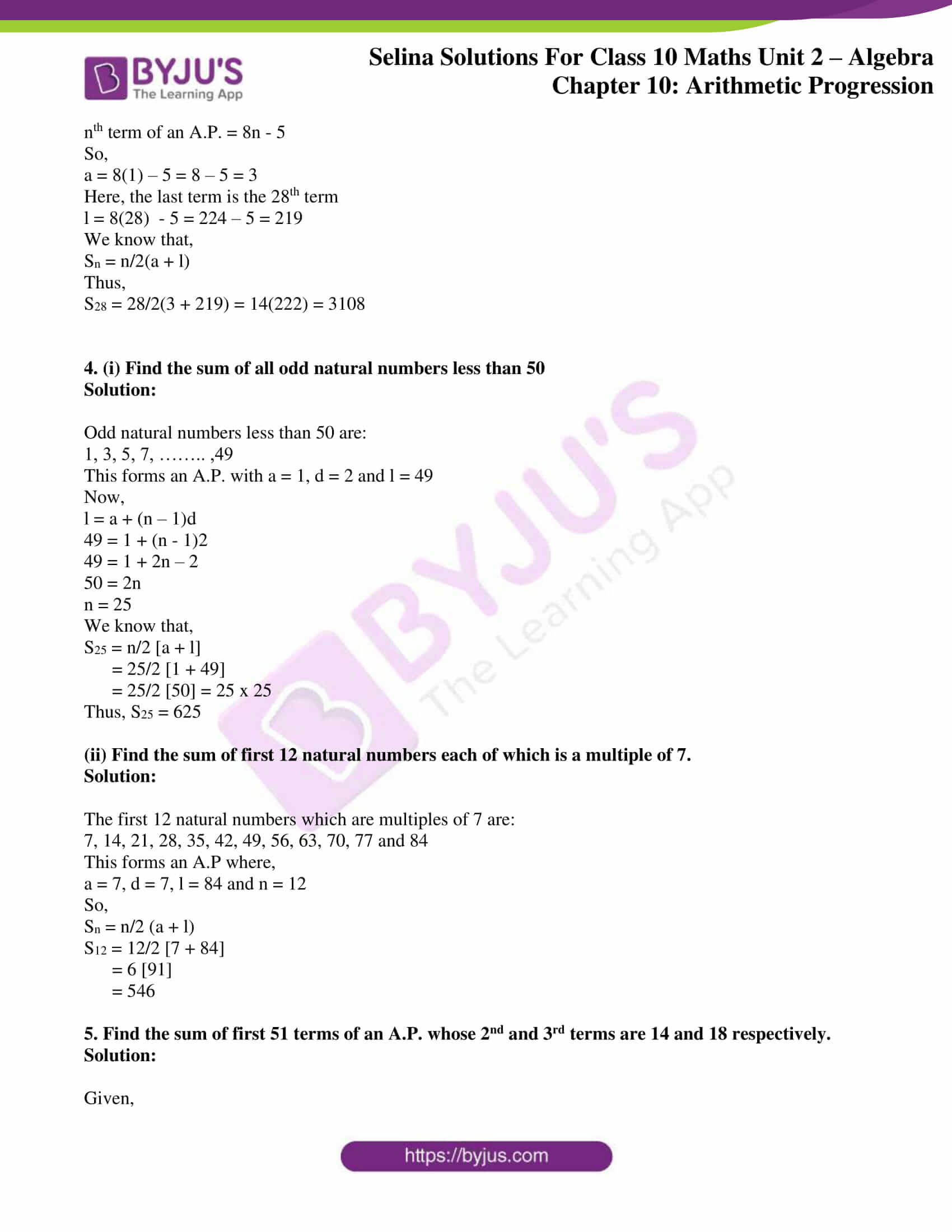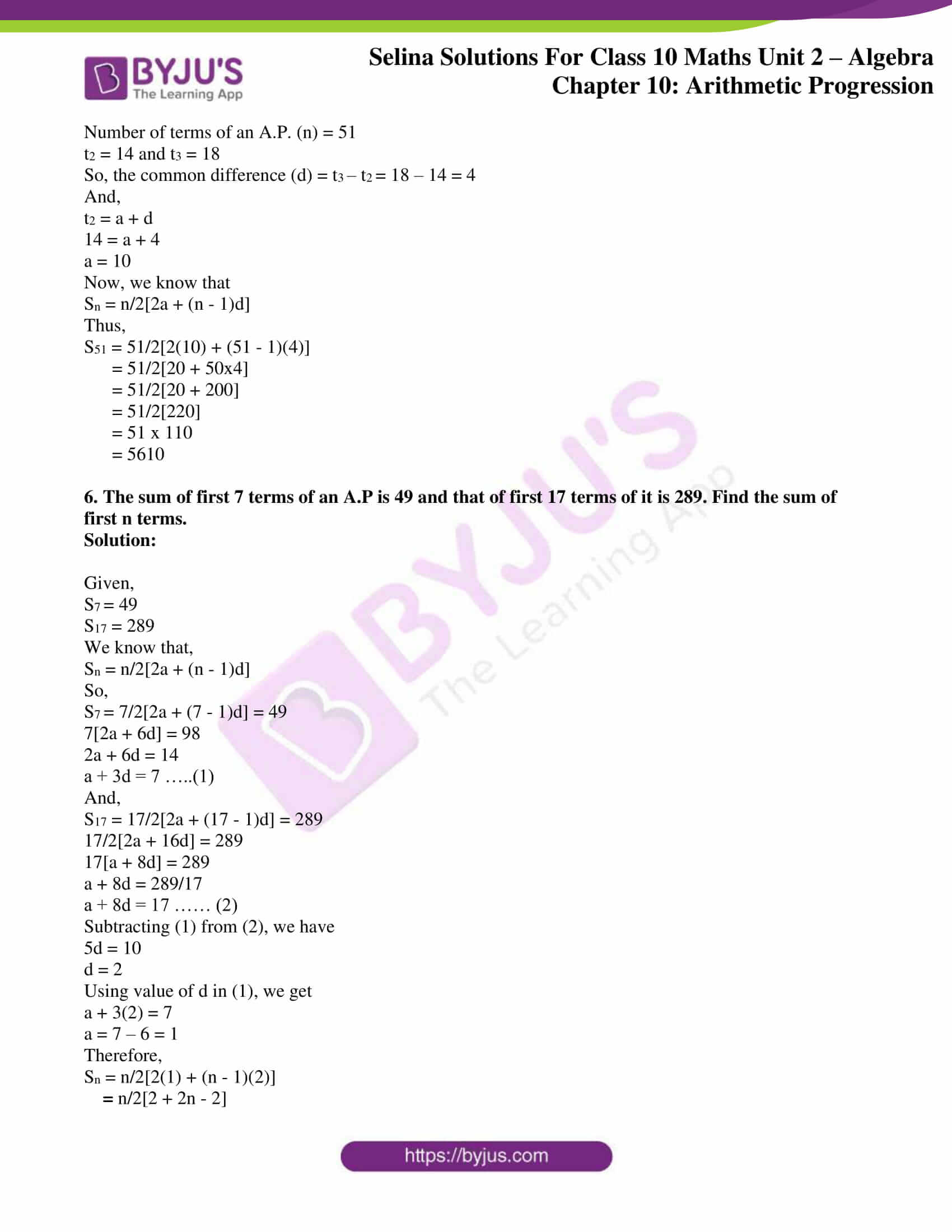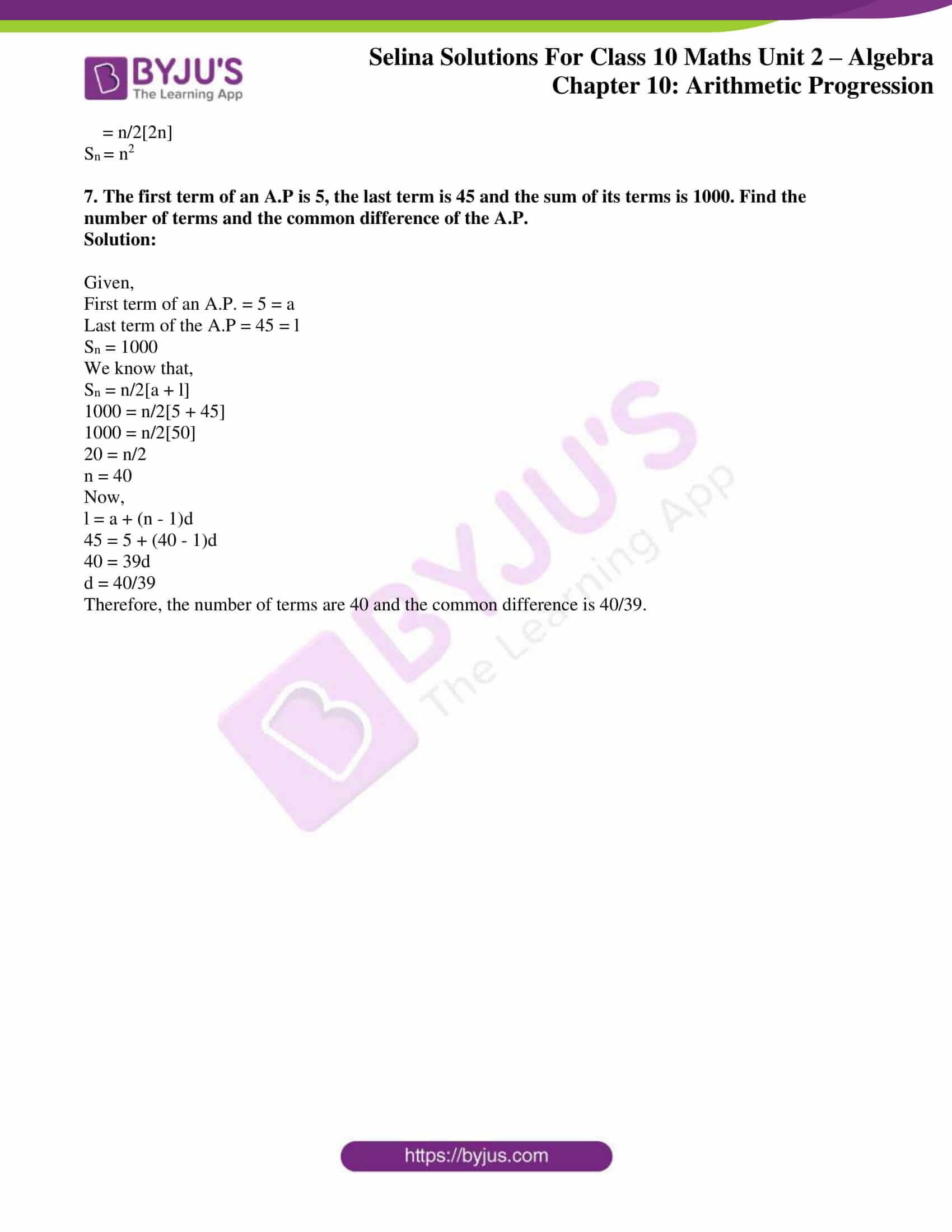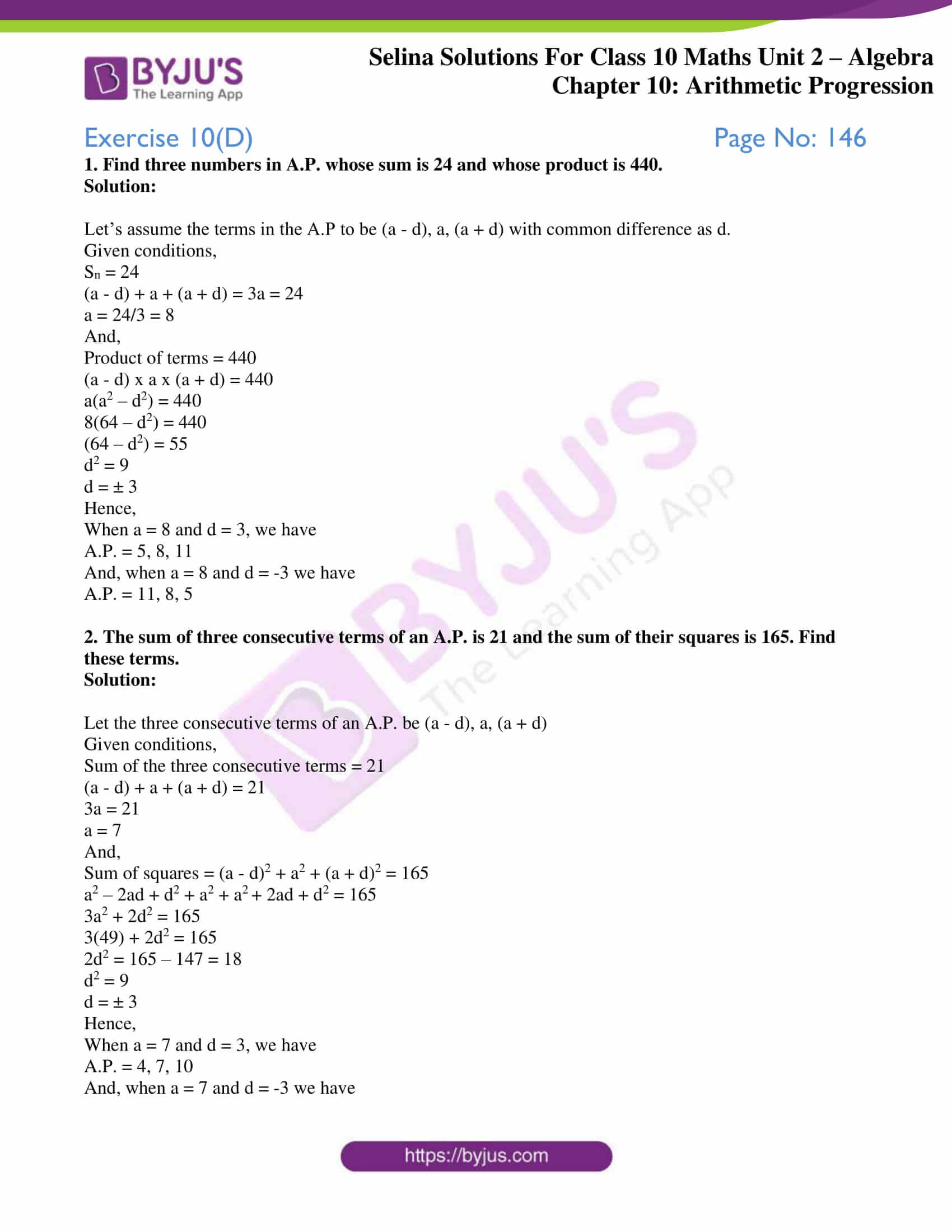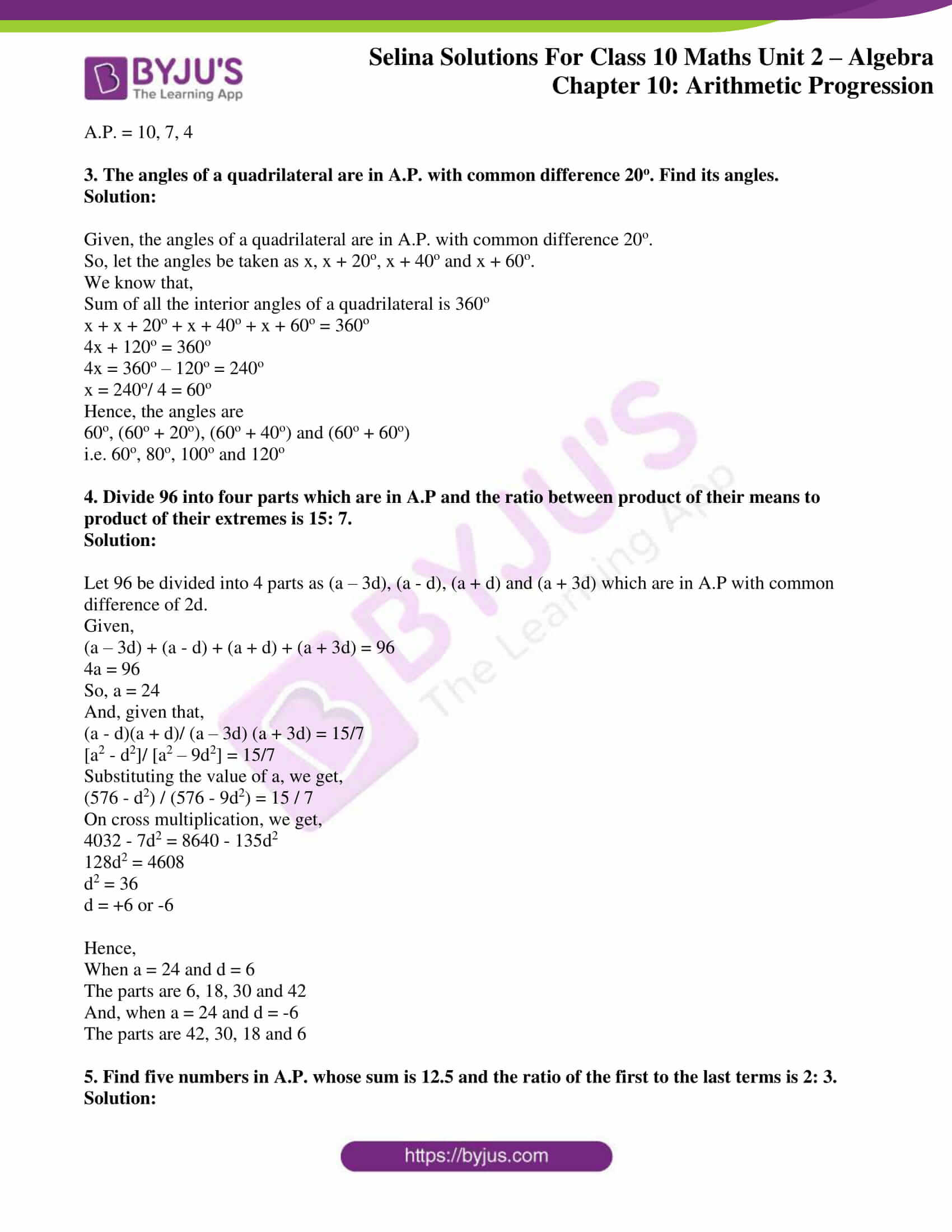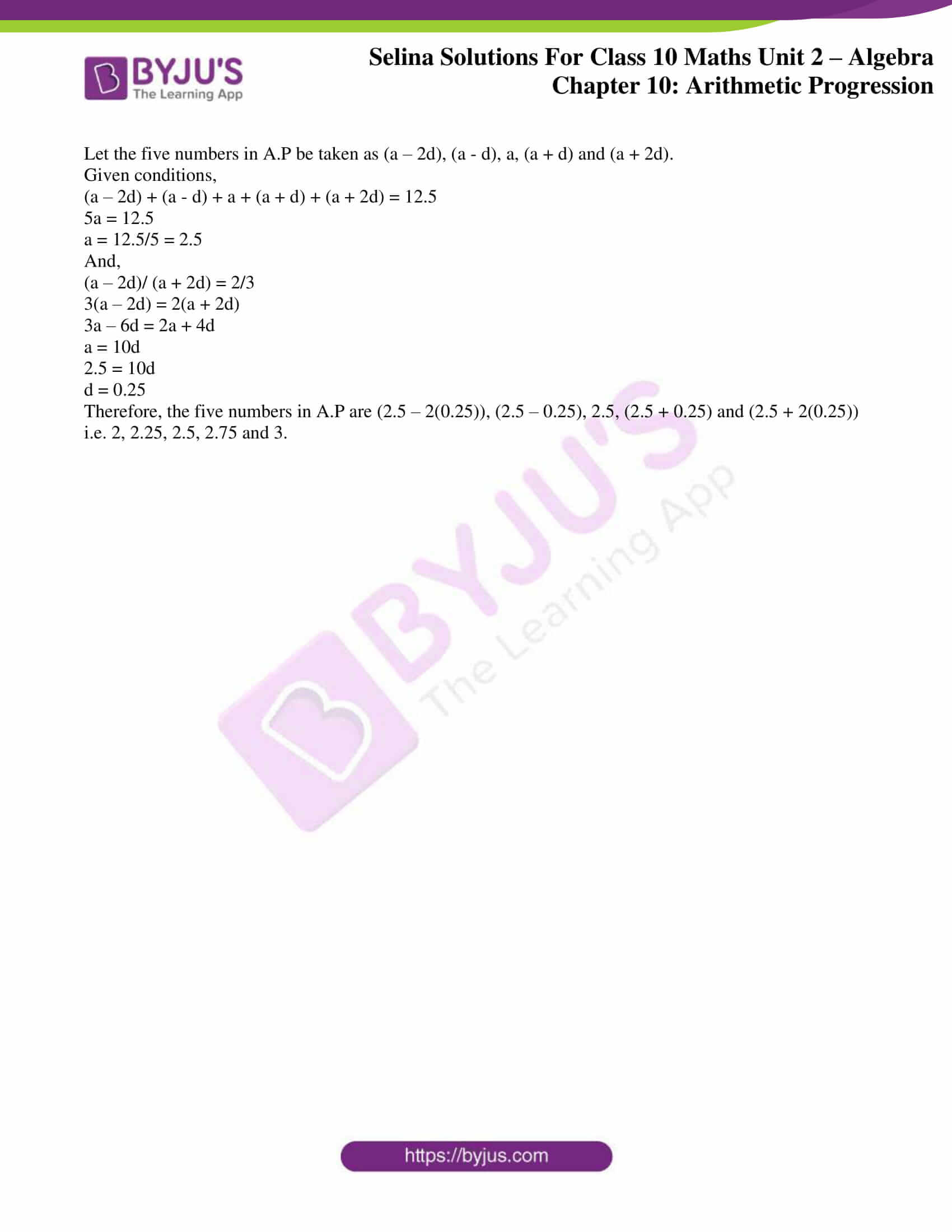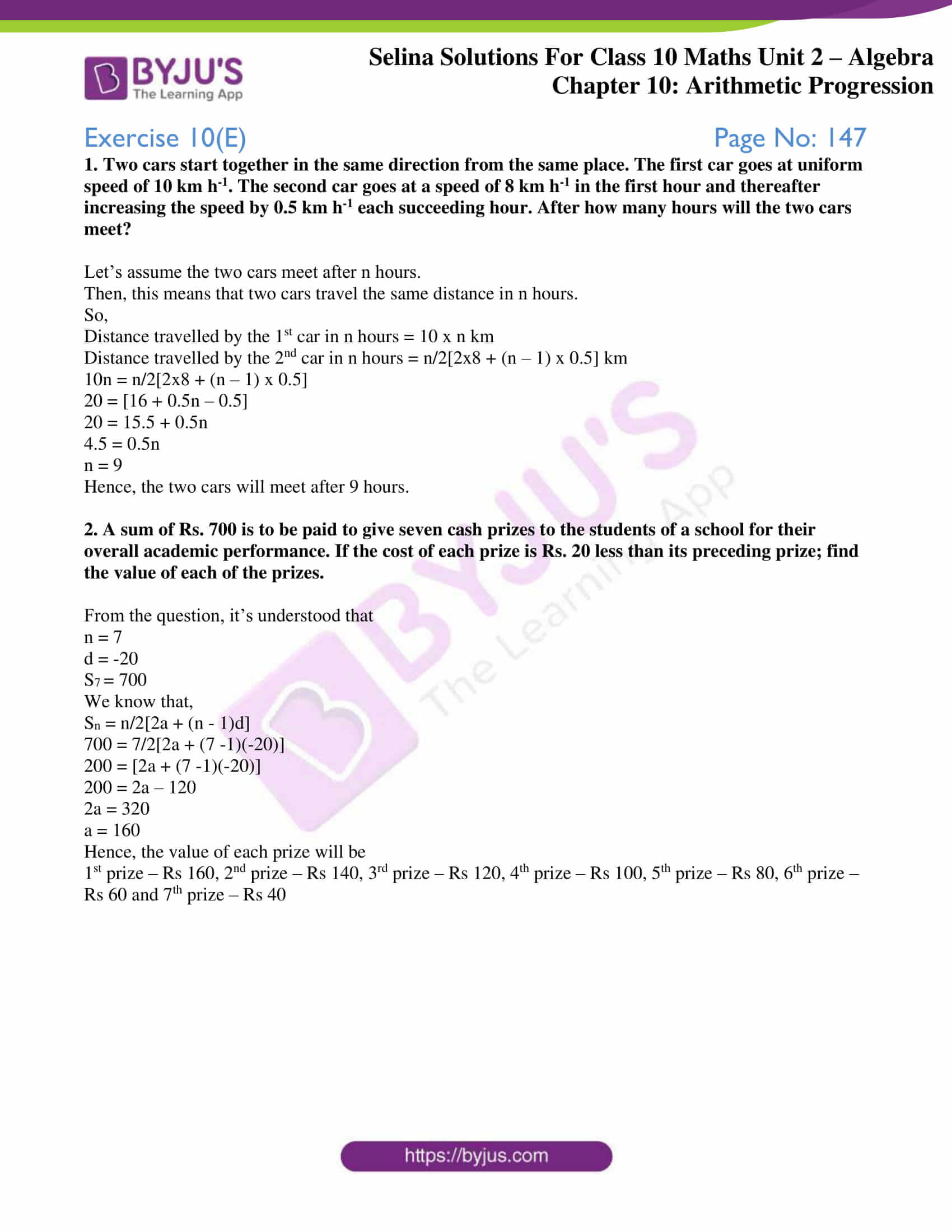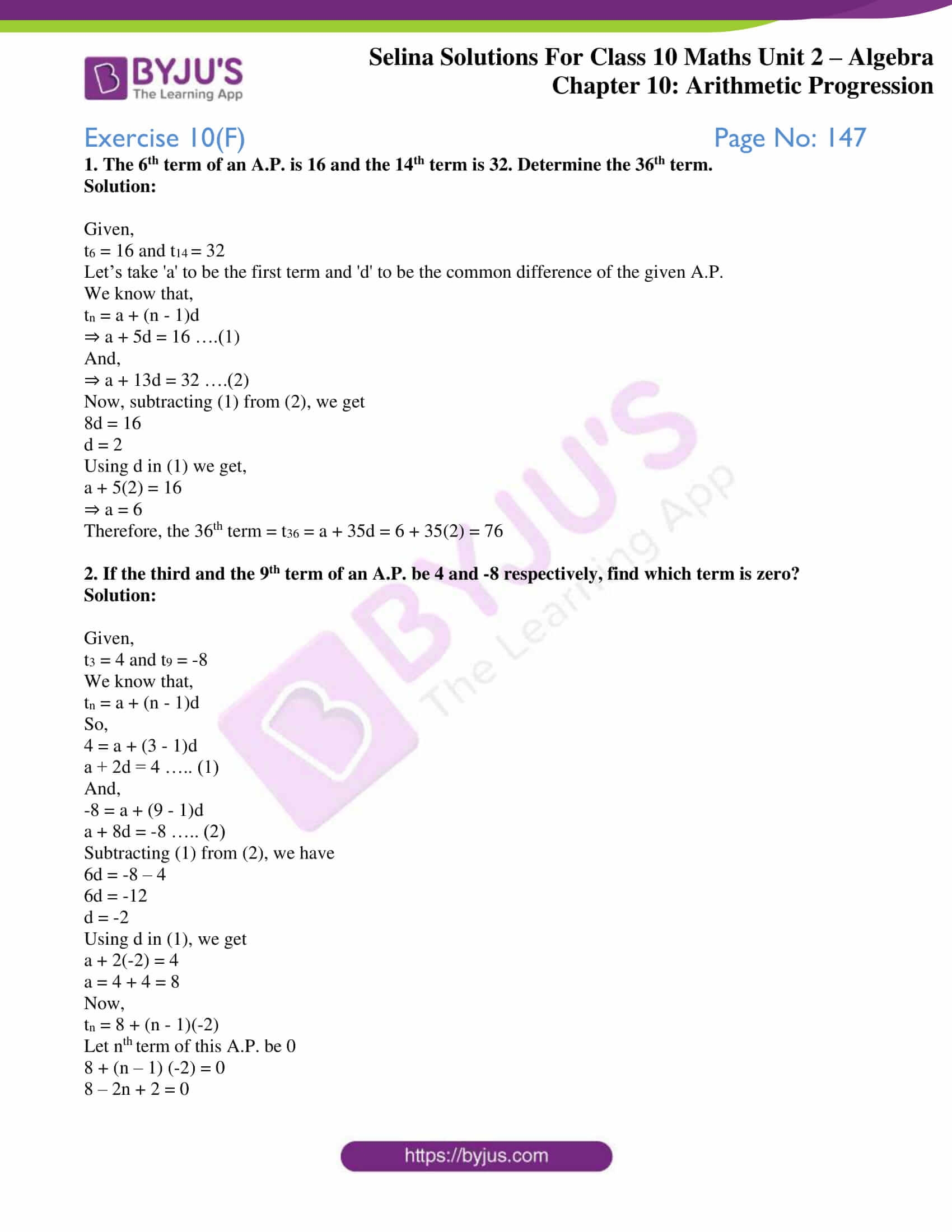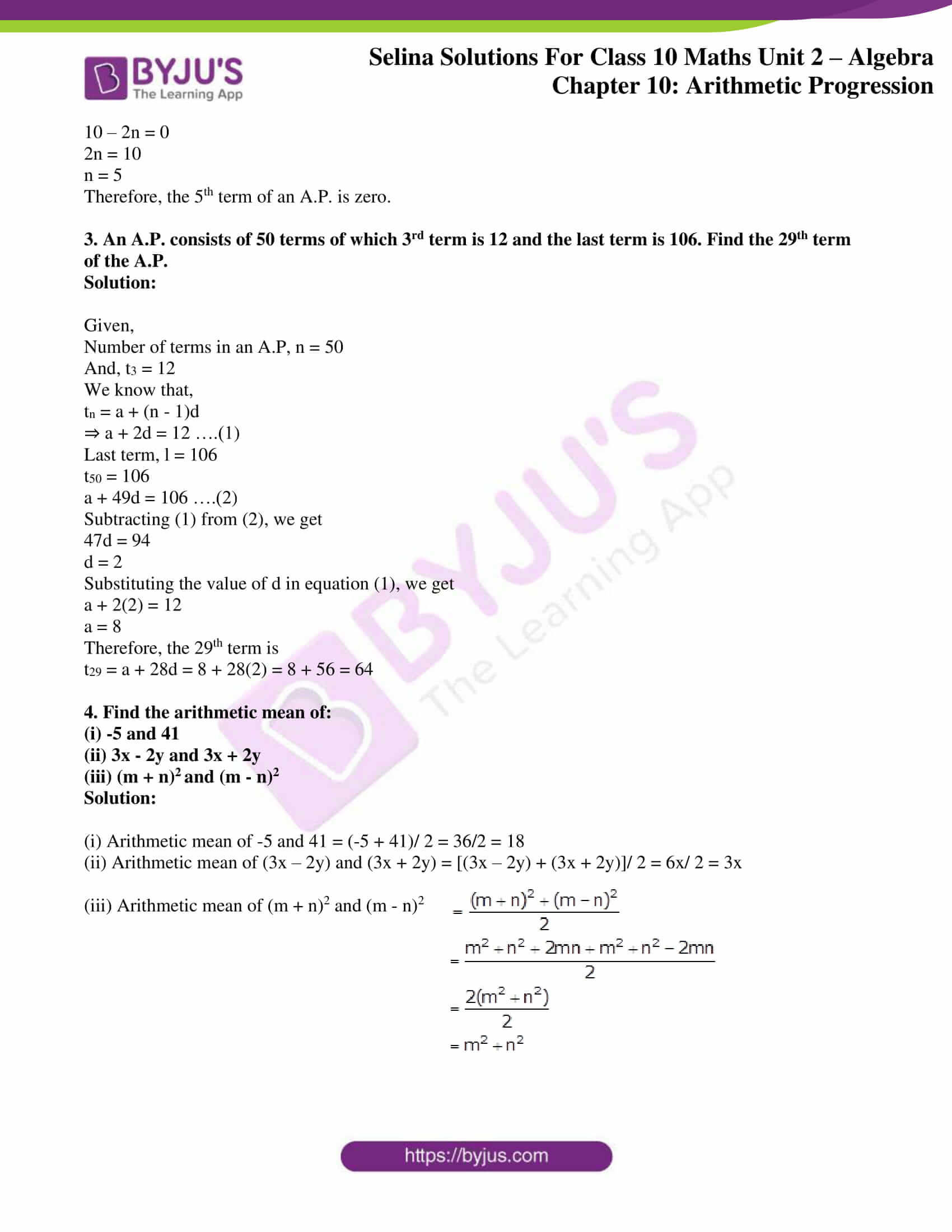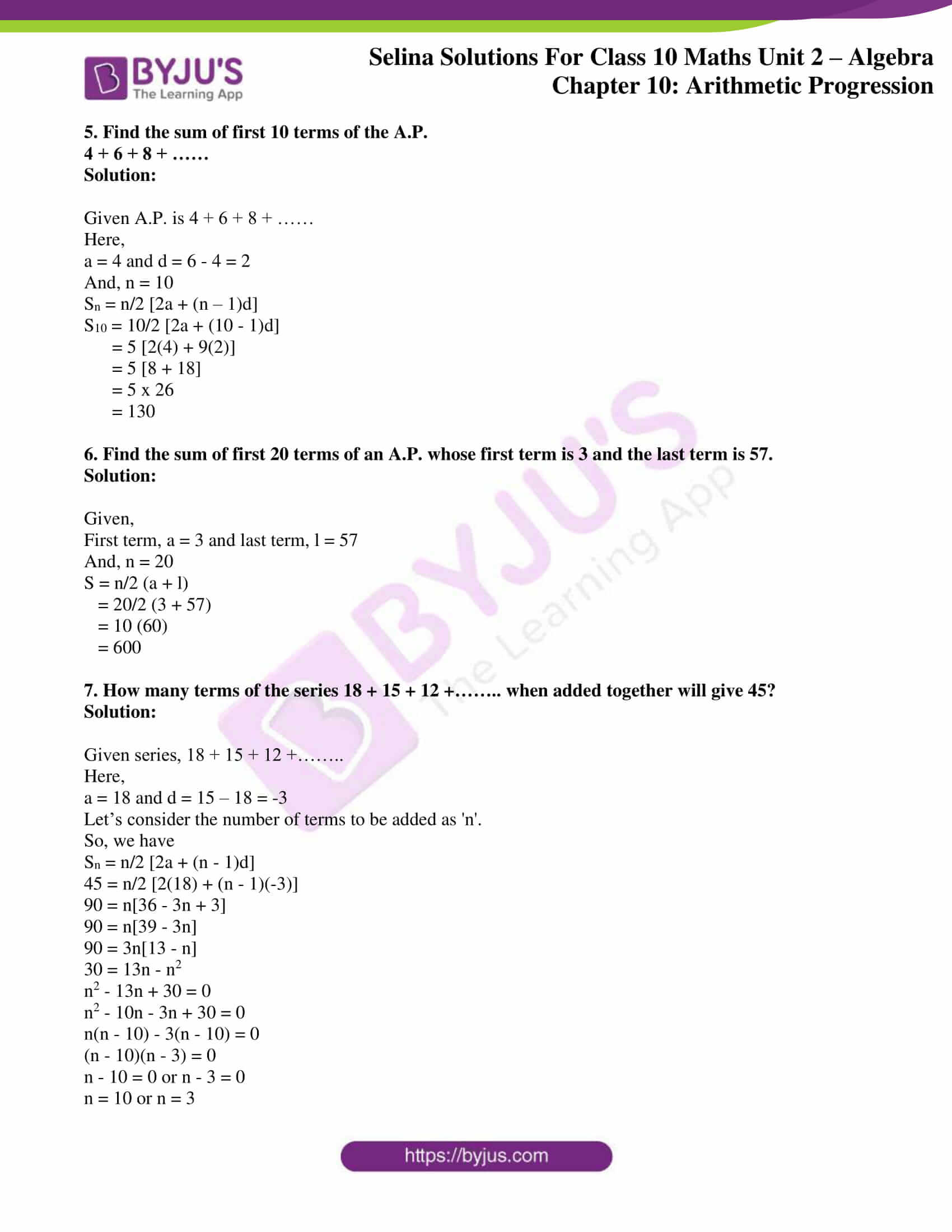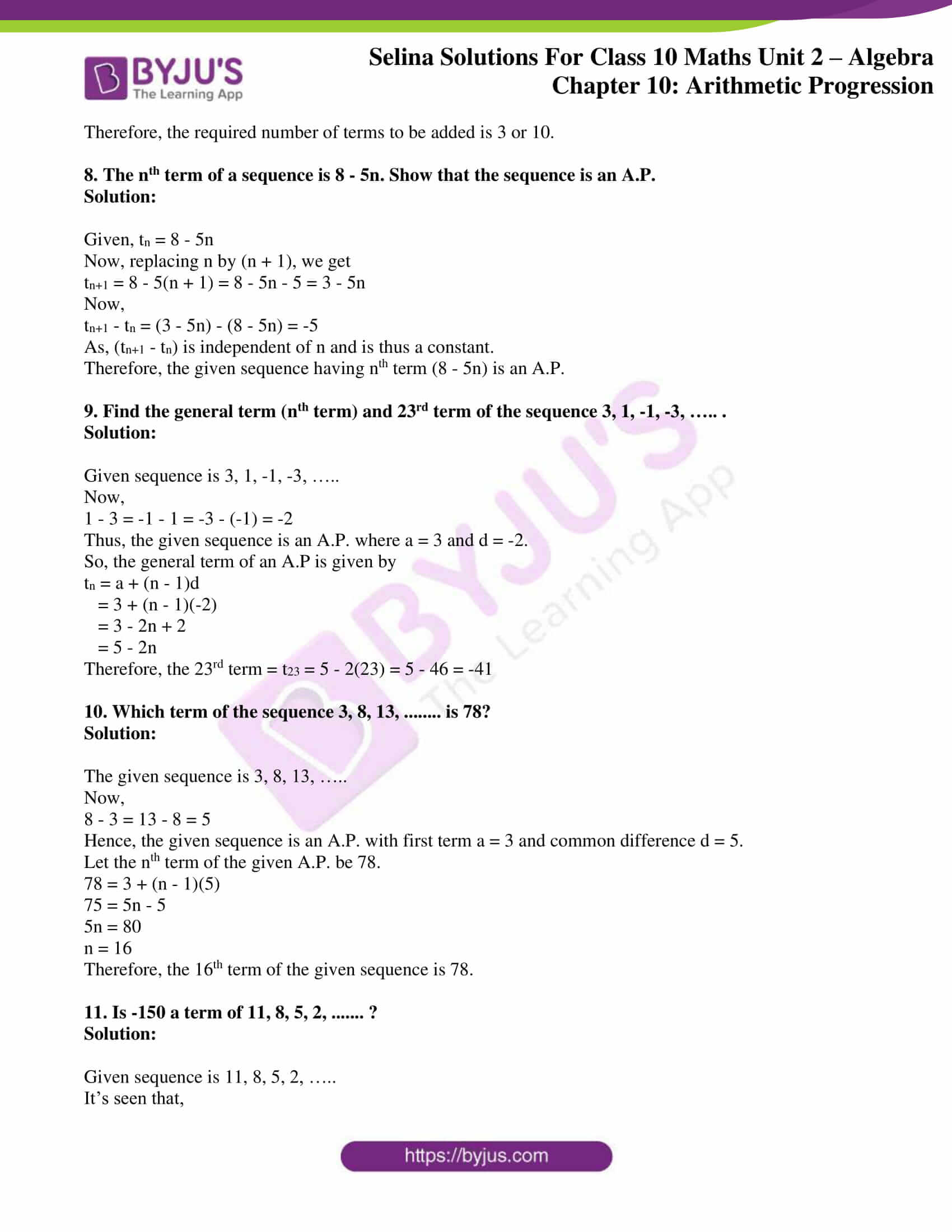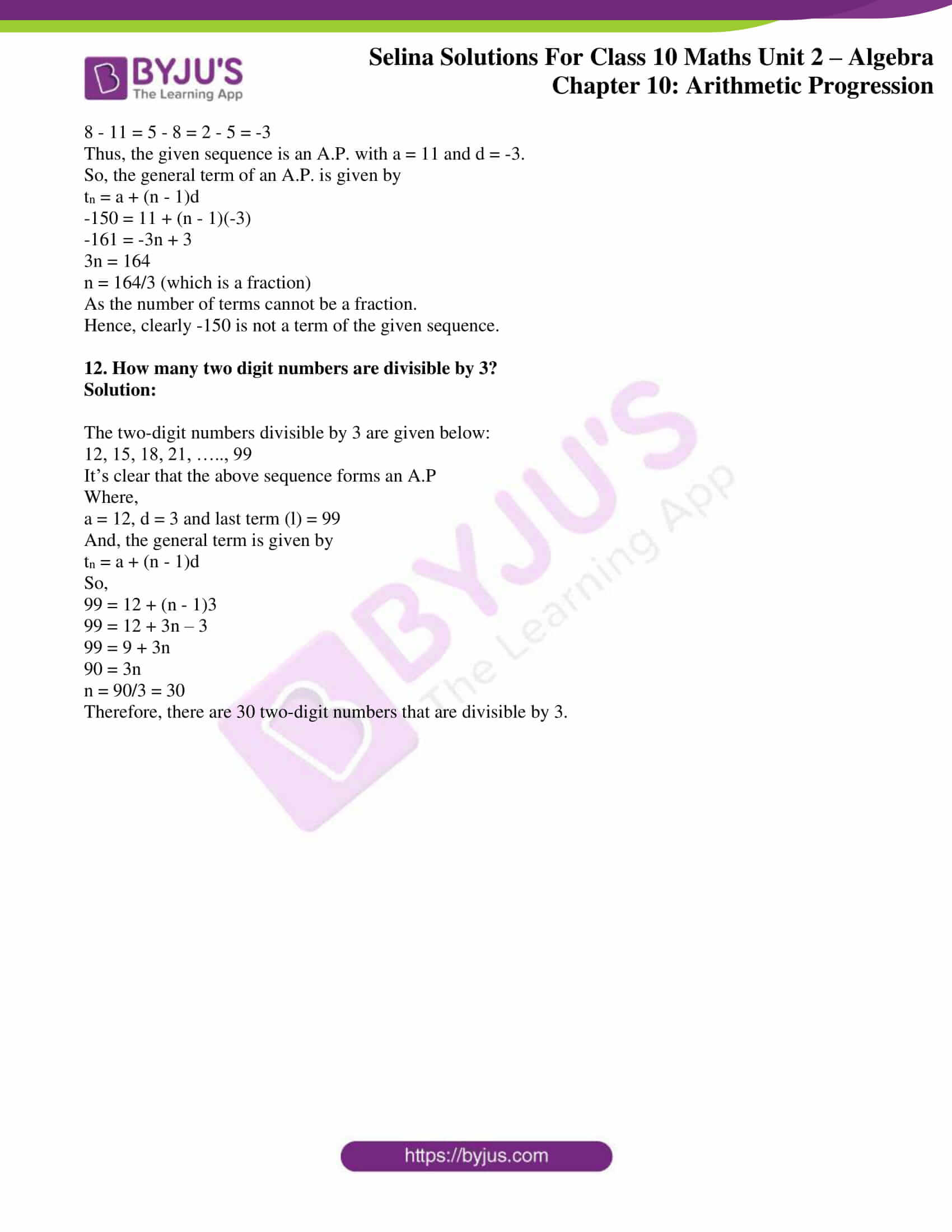## Access Selina Solutions Concise Maths Class 10 Chapter 10 Arithmetic Progression

### Exercise 10(A) Page No: 137

1. Which of the following sequences are in arithmetic progression?

(i) 2, 6, 10, 14, ….

(ii) 15, 12, 9, 6, ….

(iii) 5, 9, 12, 18, ….

(iv) 1/2, 1/3, 1/4, 1/5, ….

Solution:

(i) 2, 6, 10, 14, ….

Finding the difference between the terms,

d1 = 6 – 2 = 4

d2 = 10 – 6 = 4

d3 = 14 – 10 = 4

As d1 = d2 = d3, the given sequence is in arithmetic progression.

(ii) 15, 12, 9, 6, ….

Finding the difference between the terms,

d1 = 12 – 15 = -3

d2 = 9 – 12 = -3

d3 = 6 – 9 = -3

As d1 = d2 = d3, the given sequence is in arithmetic progression.

(iii) 5, 9, 12, 18, ….

Finding the difference between the terms,

d1 = 9 – 5 = 4

d2 = 12 – 9 = 3

d3 = 18 – 12 = 6

As d1 ≠ d2 ≠ d3, the given sequence is not in arithmetic progression.

(iv) 1/2, 1/3, 1/4, 1/5, ….

Finding the difference between the terms,

d1 = 1/3 – 1/2 = -1/6

d2 = 1/4 – 1/3 = -1/12

d3 = 1/5 – 1/4 = -1/20

As d1 ≠ d2 ≠ d3, the given sequence is not in arithmetic progression.

2. The nth term of sequence is (2n – 3), find its fifteenth term.

Solution:

Given, nth term of sequence is (2n – 3)

So, the 15th term is when n = 15

t15 = 2(15) – 3 = 30 – 3 = 27

Thus, the 15th term of the sequence is 27.

3. If the pth term of an A.P. is (2p + 3); find the A.P.

Solution:

Given, pth term of an A.P. = (2p + 3)

So, on putting p = 1, 2, 3, …, we have

t1 = 2(1) + 3 = 5

t2 = 2(2) + 3 = 7

t3 = 2(3) + 3 = 9

…..

Hence, the sequence A.P. is 5, 7, 9, …

4. Find the 24th term of the sequence:

12, 10, 8, 6,……

Solution:

Given sequence,

12, 10, 8, 6,……

The common difference:

10 – 12 = -2

8 – 10 = -2

6 – 8 = -2 ….

So, the common difference(d) of the sequence is -2 and a = 12.

Now, the general term of this A.P. is given by

tn = a + (n – 1)d = 12 + (n – 1)(-2) = 12 – 2n + 2 = 14 – 2n

For 24th term, n = 24

tn = 14 – 2(24) = 14 – 48 = -34

Therefore, the 24th term is -34

5. Find the 30th term of the sequence:

1/2, 1, 3/2, …….

Solution:

Given sequence,

1/2, 1, 3/2, …….

So,

a = ½

d = 1 – ½ = ½

We know that,

tn = a + (n – 1)d

Hence, the 30th term will be

t30 = ½ + (30 – 1)(1/2) = ½ + 29/2 = 30/2 = 15

Therefore, the 30th term is 15.

6. Find the 100th term of the sequence:

√3, 2√3, 3√3, ….

Solution:

Given A.P. is √3, 2√3, 3√3, ….

So,

a = √3

d = 2√3 – √3 = √3

The general term is given by,

tn = a + (n – 1)d

For 100th term

t100 = √3 + (100 – 1) √3 = √3 + 99√3 = 100√3

Therefore, the 100th term of the given A.P. is 100√3.

7. Find the 50th term of the sequence:

1/n, (n+1)/n, (2n+1)/n, ……

Solution:

Given sequence,

1/n, (n+1)/n, (2n+1)/n, ……

So,

a = 1/n

d = (n+1)/n – 1/n = (n+1-1)/n = 1

Then, the general term is given by

tn = a + (n – 1)d

For 50th term, n = 50

t50 = 1/n + (50 – 1)1 = 1/n + 49 = (49n + 1)/n

Hence, the 50th term of the given sequence is (49n + 1)/n.

8. Is 402 a term of the sequence: 8, 13, 18, 23,………….?

Solution:

Give sequence, 8, 13, 18, 23,………….

d = 13 – 8 = 5 and a = 8

So, the general term is given by

tn = a + (n – 1)d

tn = 8 + (n – 1)5 = 8 + 5n – 5 = 3 + 5n

Now,

If 402 is a term of the sequence whose nth is given by (3 + 5n) then n must be a non-negative integer.

3 + 5n = 402

5n = 399

n = 399/5

So, clearly n is a fraction.

Thus, we can conclude that 402 is not a term of the given sequence.

9. Find the common difference and 99th term of the arithmetic progression: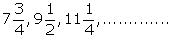Solution:Given, A.P,

i.e., 31/4, 19/2, 45/4, …….

So,

a = 31/4

Common difference, d = 19/2 – 31/4 = (38 – 31)/ 4 = 7/4

Then the general term of the A.P

tn = a + (n – 1)d

t99 = (31/4) + (99 – 1) x (7/4)

= (31/4) + 93 x (7/4)

= (31/4) + (686 / 4)

= (31 + 686)/ 4

= (717)/ 4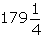= 179 ¼

Hence, the 99th term of A.P. is

10. How many terms are there in the series :

(i) 4, 7, 10, 13, …………, 148?

(ii) 0.5, 0.53, 0.56, ……………, 1.1?

(iii) 3/4, 1, 1 ¼, ……., 3?

Solution:

(i) Given series, 4, 7, 10, 13, …………, 148

Here,

a = 4 and d = 7 – 4 = 3

So, the given term is given by tn = 4 + (n – 1)3 = 4 + 3n – 3

tn = 1 + 3n

Now,

148 = 1 + 3n

147 = 3n

n = 147/3 = 49

Thus, there are 49 terms in the series.

(ii) Given series, 0.5, 0.53, 0.56, ……………, 1.1

Here,

a = 0.5 and d = 0.53 – 0.5 = 0.03

So, the given term is given by tn = 0.5 + (n – 1)0.03 = 0.5 + 0.03n – 0.03

tn = 0.47 + 0.03n

Now,

1.1 = 0.47 + 0.03n

1.1 – 0.47 = 0.03n

n = 0.63/0.03 = 21

Thus, there are 21 terms in the series.

(iii) Given series, 3/4, 1, 1 ¼, ……., 3

Here,

a = 3/4 and d = 1 – 3/4 = 1/4

So, the given term is given by tn = 3/4 + (n – 1)1/4 = (3 + n – 1)/4

tn = (2 + n)/ 4

Now,

3 = (2 + n)/ 4

12 = 2 + n

n = 10

Thus, there are 10 terms in the series.

### Exercise 10(B) Page No: 140

1. In an A.P., ten times of its tenth term is equal to thirty times of its 30th term. Find its 40th term.

Solution:

Given condition,

10 t10 = 30 t30 in an A.P.

To find: t40 = ?

We know that,

tn = a + (n – 1)d

So,

10 t10 = 30 t30

10(a + (10 – 1)d) = 30(a + (30 – 1)d)

10(a + 9d) = 30(a + 29d)

a + 9d = 3(a + 29d)

a + 9d = 3a + 87d

2a + 78d = 0

2(a + 39d) = 0

a + 39d = a + (40 – 1)d = t40 = 0

Therefore, the 40th term of the A.P. is 0

2. How many two-digit numbers are divisible by 3?

Solution:

The 2-digit numbers divisible by 3 are as follows:

12, 15, 18, 21, ………, 99

It’s seen that the above forms an A.P. with

a = 12, d = 3 and last term(nth term) = 99

We know that,

tn = a + (n – 1)d

So,

99 = 12 + (n – 1)3

99 = 12 + 3n – 3

99 = 9 + 3n

3n = 90

n = 90/3 = 30

Hence, the number of 2-digit numbers divisible by 3 is 30

3. Which term of A.P. 5, 15, 25 ………… will be 130 more than its 31st term?

Solution:

Given A.P. is 5, 15, 25, ……

a = 5, d = 10

From the question, we have

tn = t31 + 130

We know that,

tn = a + (n – 1)d

So,

5 + (n – 1)10 = 5 + (31 – 1)10 + 130

10n – 10 = 300 + 130

10n = 430 + 10 = 440

n = 440/10 = 44

Thus, the 44th term of the given A.P. is 130 more than its 31st term.

4. Find the value of p, if x, 2x + p and 3x + 6 are in A.P

Solution:

Given that,

x, 2x + p and 3x + 6 are in A.P

So, the common difference between the terms must be the same.

Hence,

2x + p – x = 3x + 6 – (2x + p)

x + p = x + 6 – p

2p = 6

p = 3

5. If the 3rd and the 9th terms of an arithmetic progression are 4 and -8 respectively, which term of it is zero?

Solution:

Given in an A.P.

t3 = 4 and t9 = -8

We know that,

tn = a + (n – 1)d

So,

a + (3 – 1)d = 4 and a + (9 – 1)d = -8

a + 2d = 4 and a + 8d = -8

Subtracting both the equations we get,

6d = -12

d = -2

Using the value of d,

a + 2(-2) = 4

a – 4 = 4

a = 8

Now,

tn = 0

8 + (n – 1)(-2) = 0

8 – 2n + 2 = 0

10 – 2n = 0

10 = 2n

n = 5

Thus, the 5th term of the A.P. is zero.

6. How many three-digit numbers are divisible by 87?

Solution:

The 3-digit numbers divisible by 87 are starting from:

174, 261, ……. , 957

This forms an A.P. with a = 174 and d = 87

And we know that,

tn = a + (n – 1)d

So,

957 = 174 + (n – 1)87

783 = (n – 1)87

(n – 1) = 9

n = 10

Thus, 10 three-digit numbers are divisible by 87.

7. For what value of n, the nth term of A.P 63, 65, 67, …….. and nth term of A.P. 3, 10, 17,…….. are equal to each other?

Solution:

Given,

A.P.1 = 63, 65, 67, ……..

a = 63, d = 2 and tn = 63 + (n – 1)2

A.P.2 = 3, 10, 17, ……..

a = 3, d = 7 and tn = 3 + (n – 1)7

Then according to the question,

nth of A.P.1 = nth of A.P.2

63 + (n – 1)2 = 3 + (n – 1)7

60 + 2n – 2 = 7n – 7

58 + 7 = 5n

n = 65/5 = 13

Therefore, the 13th term of both the A.P.s is equal to each other.

8. Determine the A.P. whose 3rd term is 16 and the 7th term exceeds the 5th term by 12.

Solution:

Given,

t3 of an A.P. = 16 and

t7 = t5 + 12

We know that,

tn = a + (n – 1)d

So,

t3 = a + (3 – 1)d = 16

a + 2d = 16 …… (i)

And,

a + (7 – 1)d = a + (5 – 1)d + 12

6d = 4d + 12

2d = 12

d = 6

Using ‘d’ in (i) we get,

a + 2(6) = 16

a + 12 = 16

a = 4

Hence, after finding the first term = 4 and common difference = 6 the A.P. can be formed.

i.e. 4, 10, 16, 22, 28, …….

### Exercise 10(C) Page No: 143

1. Find the sum of the first 22 terms of the A.P.: 8, 3, -2, ………..

Solution:

Given, A.P. is 8, 3, -2, ………..

Here,

a = 8

d = 3 – 8 = -5

And we know that,

Sn = n/2[2a + (n – 1)d]

So,

S22 = 22/2[2(8) + (22 – 1)(-5)]

= 11[16 + (21 x -5)]

= 11[16 – 105]

= 11[-89]

= – 979

2. How many terms of the A.P. :

24, 21, 18, ……… must be taken so that their sum is 78?

Solution:

Given, A.P. is 24, 21, 18, ………

Here,

a = 24

d = 21 – 24 = -3 and

Sn = 78

We know that,

Sn = n/2[2a + (n – 1)d]

So,

78 = n/2[2(24) + (n – 1)(-3)]

78 = n/2[48 – 3n + 3]

156 = n[51 – 3n]

3n2 – 51n + 156 = 0

n2 – 17n + 52 = 0

n2 – 13n – 4n + 52 = 0

n(n – 13) – 4(n – 13) = 0

(n – 4) (n – 13) = 0

So, n – 4 = 0 or n – 13 = 0

Thus, n = 4 or 13

Hence, the required number of terms to be taken can be first 4 or first 13.

3. Find the sum of 28 terms of an A.P. whose nth term is 8n – 5.

Solution:

Given,

nth term of an A.P. = 8n – 5

So,

a = 8(1) – 5 = 8 – 5 = 3

Here, the last term is the 28th term

l = 8(28) – 5 = 224 – 5 = 219

We know that,

Sn = n/2(a + l)

Thus,

S28 = 28/2(3 + 219) = 14(222) = 3108

4. (i) Find the sum of all odd natural numbers less than 50

Solution:

Odd natural numbers less than 50 are:

1, 3, 5, 7, …….. ,49

This forms an A.P. with a = 1, d = 2 and l = 49

Now,

l = a + (n – 1)d

49 = 1 + (n – 1)2

49 = 1 + 2n – 2

50 = 2n

n = 25

We know that,

S25 = n/2 [a + l]

= 25/2 [1 + 49]

= 25/2  = 25 x 25

Thus, S25 = 625

(ii) Find the sum of first 12 natural numbers each of which is a multiple of 7.

Solution:

The first 12 natural numbers which are multiples of 7 are:

7, 14, 21, 28, 35, 42, 49, 56, 63, 70, 77 and 84

This forms an A.P where,

a = 7, d = 7, l = 84 and n = 12

So,

Sn = n/2 (a + l)

S12 = 12/2 [7 + 84]

= 6 

= 546

5. Find the sum of first 51 terms of an A.P. whose 2nd and 3rd terms are 14 and 18 respectively.

Solution:

Given,

Number of terms of an A.P. (n) = 51

t2 = 14 and t3 = 18

So, the common difference (d) = t3 – t2 = 18 – 14 = 4

And,

t2 = a + d

14 = a + 4

a = 10

Now, we know that

Sn = n/2[2a + (n – 1)d]

Thus,

S51 = 51/2[2(10) + (51 – 1)(4)]

= 51/2[20 + 50×4]

= 51/2[20 + 200]

= 51/2

= 51 x 110

= 5610

6. The sum of first 7 terms of an A.P is 49 and that of first 17 terms of it is 289. Find the sum of first n terms.

Solution:

Given,

S7 = 49

S17 = 289

We know that,

Sn = n/2[2a + (n – 1)d]

So,

S7 = 7/2[2a + (7 – 1)d] = 49

7[2a + 6d] = 98

2a + 6d = 14

a + 3d = 7 …..(1)

And,

S17 = 17/2[2a + (17 – 1)d] = 289

17/2[2a + 16d] = 289

17[a + 8d] = 289

a + 8d = 289/17

a + 8d = 17 …… (2)

Subtracting (1) from (2), we have

5d = 10

d = 2

Using value of d in (1), we get

a + 3(2) = 7

a = 7 – 6 = 1

Therefore,

Sn = n/2[2(1) + (n – 1)(2)]

= n/2[2 + 2n – 2]

= n/2[2n]

Sn = n2

7. The first term of an A.P is 5, the last term is 45 and the sum of its terms is 1000. Find the number of terms and the common difference of the A.P.

Solution:

Given,

First term of an A.P. = 5 = a

Last term of the A.P = 45 = l

Sn = 1000

We know that,

Sn = n/2[a + l]

1000 = n/2[5 + 45]

1000 = n/2

20 = n/2

n = 40

Now,

l = a + (n – 1)d

45 = 5 + (40 – 1)d

40 = 39d

d = 40/39

Therefore, the number of terms are 40 and the common difference is 40/39.

### Exercise 10(D) Page No: 146

1. Find three numbers in A.P. whose sum is 24 and whose product is 440.

Solution:

Let’s assume the terms in the A.P to be (a – d), a, (a + d) with common difference as d.

Given conditions,

Sn = 24

(a – d) + a + (a + d) = 3a = 24

a = 24/3 = 8

And,

Product of terms = 440

(a – d) x a x (a + d) = 440

a(a2 – d2) = 440

8(64 – d2) = 440

(64 – d2) = 55

d2 = 9

d = ± 3

Hence,

When a = 8 and d = 3, we have

A.P. = 5, 8, 11

And, when a = 8 and d = -3 we have

A.P. = 11, 8, 5

2. The sum of three consecutive terms of an A.P. is 21 and the sum of their squares is 165. Find these terms.

Solution:

Let the three consecutive terms of an A.P. be (a – d), a, (a + d)

Given conditions,

Sum of the three consecutive terms = 21

(a – d) + a + (a + d) = 21

3a = 21

a = 7

And,

Sum of squares = (a – d)2 + a2 + (a + d)2 = 165

a2 – 2ad + d2 + a2 + a2 + 2ad + d2 = 165

3a2 + 2d2 = 165

3(49) + 2d2 = 165

2d2 = 165 – 147 = 18

d2 = 9

d = ± 3

Hence,

When a = 7 and d = 3, we have

A.P. = 4, 7, 10

And, when a = 7 and d = -3 we have

A.P. = 10, 7, 4

3. The angles of a quadrilateral are in A.P. with common difference 20o. Find its angles.

Solution:

Given, the angles of a quadrilateral are in A.P. with common difference 20o.

So, let the angles be taken as x, x + 20o, x + 40o and x + 60o.

We know that,

Sum of all the interior angles of a quadrilateral is 360o

x + x + 20o + x + 40o + x + 60o = 360o

4x + 120o = 360o

4x = 360o – 120o = 240o

x = 240o/ 4 = 60o

Hence, the angles are

60o, (60o + 20o), (60o + 40o) and (60o + 60o)

i.e. 60o, 80o, 100o and 120o

4. Divide 96 into four parts which are in A.P and the ratio between product of their means to product of their extremes is 15: 7.

Solution:

Let 96 be divided into 4 parts as (a – 3d), (a – d), (a + d) and (a + 3d) which are in A.P with common difference of 2d.

Given,

(a – 3d) + (a – d) + (a + d) + (a + 3d) = 96

4a = 96

So, a = 24

And, given that,

(a – d)(a + d)/ (a – 3d) (a + 3d) = 15/7

[a2 – d2]/ [a2 – 9d2] = 15/7

Substituting the value of a, we get,

(576 – d2) / (576 – 9d2) = 15 / 7

On cross multiplication, we get,

4032 – 7d2 = 8640 – 135d2

128d2 = 4608

d2 = 36

d = +6 or -6

Hence,

When a = 24 and d = 6

The parts are 6, 18, 30 and 42

And, when a = 24 and d = -6

The parts are 42, 30, 18 and 6

5. Find five numbers in A.P. whose sum is 12.5 and the ratio of the first to the last terms is 2: 3.

Solution:

Let the five numbers in A.P be taken as (a – 2d), (a – d), a, (a + d) and (a + 2d).

Given conditions,

(a – 2d) + (a – d) + a + (a + d) + (a + 2d) = 12.5

5a = 12.5

a = 12.5/5 = 2.5

And,

(a – 2d)/ (a + 2d) = 2/3

3(a – 2d) = 2(a + 2d)

3a – 6d = 2a + 4d

a = 10d

2.5 = 10d

d = 0.25

Therefore, the five numbers in A.P are (2.5 – 2(0.25)), (2.5 – 0.25), 2.5, (2.5 + 0.25) and (2.5 + 2(0.25))

i.e. 2, 2.25, 2.5, 2.75 and 3.

### Exercise 10(E) Page No: 147

1. Two cars start together in the same direction from the same place. The first car goes at uniform speed of 10 km h-1. The second car goes at a speed of 8 km h-1 in the first hour and thereafter increasing the speed by 0.5 km h-1 each succeeding hour. After how many hours will the two cars meet?

Let’s assume the two cars meet after n hours.

Then, this means that two cars travel the same distance in n hours.

So,

Distance travelled by the 1st car in n hours = 10 x n km

Distance travelled by the 2nd car in n hours = n/2[2×8 + (n – 1) x 0.5] km

10n = n/2[2×8 + (n – 1) x 0.5]

20 = [16 + 0.5n – 0.5]

20 = 15.5 + 0.5n

4.5 = 0.5n

n = 9

Hence, the two cars will meet after 9 hours.

2. A sum of Rs. 700 is to be paid to give seven cash prizes to the students of a school for their overall academic performance. If the cost of each prize is Rs. 20 less than its preceding prize; find the value of each of the prizes.

From the question, it’s understood that

n = 7

d = -20

S7 = 700

We know that,

Sn = n/2[2a + (n – 1)d]

700 = 7/2[2a + (7 -1)(-20)]

200 = [2a + (7 -1)(-20)]

200 = 2a – 120

2a = 320

a = 160

Hence, the value of each prize will be

1st prize – Rs 160, 2nd prize – Rs 140, 3rd prize – Rs 120, 4th prize – Rs 100, 5th prize – Rs 80, 6th prize – Rs 60 and 7th prize – Rs 40

### Exercise 10(F) Page No: 147

1. The 6th term of an A.P. is 16 and the 14th term is 32. Determine the 36th term.

Solution:

Given,

t6 = 16 and t14 = 32

Let’s take ‘a’ to be the first term and ‘d’ to be the common difference of the given A.P.

We know that,

tn = a + (n – 1)d

⇒ a + 5d = 16 ….(1)

And,

⇒ a + 13d = 32 ….(2)

Now, subtracting (1) from (2), we get

8d = 16

d = 2

Using d in (1) we get,

a + 5(2) = 16

⇒ a = 6

Therefore, the 36th term = t36 = a + 35d = 6 + 35(2) = 76

2. If the third and the 9th term of an A.P. be 4 and -8 respectively, find which term is zero?

Solution:

Given,

t3 = 4 and t9 = -8

We know that,

tn = a + (n – 1)d

So,

4 = a + (3 – 1)d

a + 2d = 4 ….. (1)

And,

-8 = a + (9 – 1)d

a + 8d = -8 ….. (2)

Subtracting (1) from (2), we have

6d = -8 – 4

6d = -12

d = -2

Using d in (1), we get

a + 2(-2) = 4

a = 4 + 4 = 8

Now,

tn = 8 + (n – 1)(-2)

Let nth term of this A.P. be 0

8 + (n – 1) (-2) = 0

8 – 2n + 2 = 0

10 – 2n = 0

2n = 10

n = 5

Therefore, the 5th term of an A.P. is zero.

3. An A.P. consists of 50 terms of which 3rd term is 12 and the last term is 106. Find the 29th term of the A.P.

Solution:

Given,

Number of terms in an A.P, n = 50

And, t3 = 12

We know that,

tn = a + (n – 1)d

⇒ a + 2d = 12 ….(1)

Last term, l = 106

t50 = 106

a + 49d = 106 ….(2)

Subtracting (1) from (2), we get

47d = 94

d = 2

Substituting the value of d in equation (1), we get

a + 2(2) = 12

a = 8

Therefore, the 29th term is

t29 = a + 28d = 8 + 28(2) = 8 + 56 = 64

4. Find the arithmetic mean of:

(i) -5 and 41

(ii) 3x – 2y and 3x + 2y

(iii) (m + n)and (m – n)2

Solution:

(i) Arithmetic mean of -5 and 41 = (-5 + 41)/ 2 = 36/2 = 18

(ii) Arithmetic mean of (3x – 2y) and (3x + 2y) = [(3x – 2y) + (3x + 2y)]/ 2 = 6x/ 2 = 3x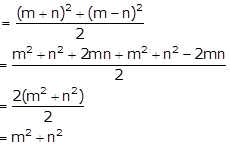(iii) Arithmetic mean of (m + n)2 and (m – n)2

5. Find the sum of first 10 terms of the A.P.

4 + 6 + 8 + ……

Solution:

Given A.P. is 4 + 6 + 8 + ……

Here,

a = 4 and d = 6 – 4 = 2

And, n = 10

Sn = n/2 [2a + (n – 1)d]

S10 = 10/2 [2a + (10 – 1)d]

= 5 [2(4) + 9(2)]

= 5 [8 + 18]

= 5 x 26

= 130

6. Find the sum of first 20 terms of an A.P. whose first term is 3 and the last term is 57.

Solution:

Given,

First term, a = 3 and last term, l = 57

And, n = 20

S = n/2 (a + l)

= 20/2 (3 + 57)

= 10 (60)

= 600

7. How many terms of the series 18 + 15 + 12 +…….. when added together will give 45?

Solution:

Given series, 18 + 15 + 12 +……..

Here,

a = 18 and d = 15 – 18 = -3

Let’s consider the number of terms to be added as ‘n’.

So, we have

Sn = n/2 [2a + (n – 1)d]

45 = n/2 [2(18) + (n – 1)(-3)]

90 = n[36 – 3n + 3]

90 = n[39 – 3n]

90 = 3n[13 – n]

30 = 13n – n2

n2 – 13n + 30 = 0

n2 – 10n – 3n + 30 = 0

n(n – 10) – 3(n – 10) = 0

(n – 10)(n – 3) = 0

n – 10 = 0 or n – 3 = 0

n = 10 or n = 3

Therefore, the required number of terms to be added is 3 or 10.

8. The nth term of a sequence is 8 – 5n. Show that the sequence is an A.P.

Solution:

Given, tn = 8 – 5n

Now, replacing n by (n + 1), we get

tn+1 = 8 – 5(n + 1) = 8 – 5n – 5 = 3 – 5n

Now,

tn+1 – tn = (3 – 5n) – (8 – 5n) = -5

As, (tn+1 – tn) is independent of n and is thus a constant.

Therefore, the given sequence having nth term (8 – 5n) is an A.P.

9. Find the general term (nth term) and 23rd term of the sequence 3, 1, -1, -3, ….. .

Solution:

Given sequence is 3, 1, -1, -3, …..

Now,

1 – 3 = -1 – 1 = -3 – (-1) = -2

Thus, the given sequence is an A.P. where a = 3 and d = -2.

So, the general term of an A.P is given by

tn = a + (n – 1)d

= 3 + (n – 1)(-2)

= 3 – 2n + 2

= 5 – 2n

Therefore, the 23rd term = t23 = 5 – 2(23) = 5 – 46 = -41

10. Which term of the sequence 3, 8, 13, …….. is 78?

Solution:

The given sequence is 3, 8, 13, …..

Now,

8 – 3 = 13 – 8 = 5

Hence, the given sequence is an A.P. with first term a = 3 and common difference d = 5.

Let the nth term of the given A.P. be 78.

78 = 3 + (n – 1)(5)

75 = 5n – 5

5n = 80

n = 16

Therefore, the 16th term of the given sequence is 78.

11. Is -150 a term of 11, 8, 5, 2, ……. ?

Solution:

Given sequence is 11, 8, 5, 2, …..

It’s seen that,

8 – 11 = 5 – 8 = 2 – 5 = -3

Thus, the given sequence is an A.P. with a = 11 and d = -3.

So, the general term of an A.P. is given by

tn = a + (n – 1)d

-150 = 11 + (n – 1)(-3)

-161 = -3n + 3

3n = 164

n = 164/3 (which is a fraction)

As the number of terms cannot be a fraction.

Hence, clearly -150 is not a term of the given sequence.

12. How many two digit numbers are divisible by 3?

Solution:

The two-digit numbers divisible by 3 are given below:

12, 15, 18, 21, ….., 99

It’s clear that the above sequence forms an A.P

Where,

a = 12, d = 3 and last term (l) = 99

And, the general term is given by

tn = a + (n – 1)d

So,

99 = 12 + (n – 1)3

99 = 12 + 3n – 3

99 = 9 + 3n

90 = 3n

n = 90/3 = 30

Therefore, there are 30 two-digit numbers that are divisible by 3.

### Exercises of Selina Solutions Concise Maths Class 10 Chapter 10 Arithmetic Progression

Exercise 10(A) Solutions

Exercise 10(B) Solutions

Exercise 10(C) Solutions

Exercise 10(D) Solutions

Exercise 10(E) Solutions

Exercise 10(F) Solutions